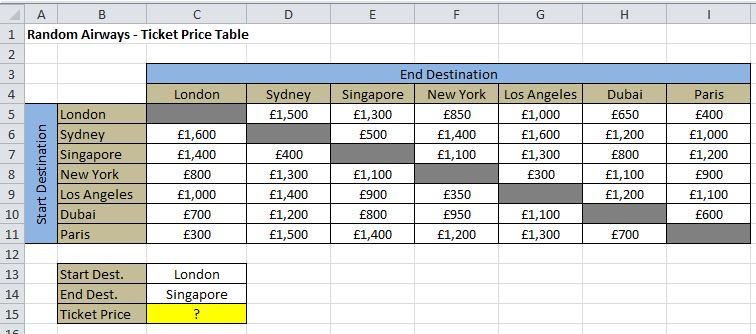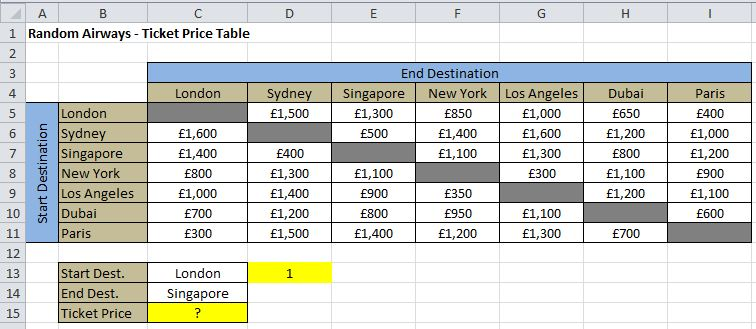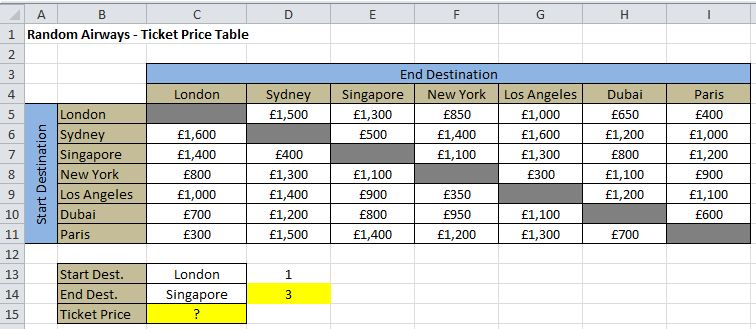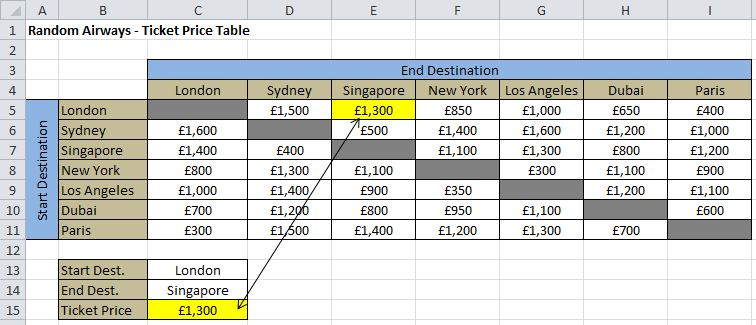# How to lookup a value in Excel based on the row and column

There are times as a Data analyst where you will need to lookup a value in a table based on the row and column, or based on two values. This can seem tricky at first if you do not know the correct approach or functions to use so read on to find out how to use Excels index and match functions to find a value in a table based off two criteria’s.

Scenario

Your manager emails you the following spreadsheet which contains ticket prices for various flights at Random Airways, she wants to be able to enter the start destination and the end destination and for Excel to return the ticket price from that table:As you can see this will require matching a start destination and an End destination and returning the value based on those two locations. Effectively this translates in Excel to looking up a row number, a column number and then finding the value where those two points cross, also known as the intercept.

Approach

The best way to approach Excel tasks that are slightly more complicated than standard is to break the problem down into smaller parts, solve the parts and then put everything back together at the end (this is also a great tip for large multiple IF statements).

If we break the problem down there are 3 distinct parts to solve:

1) Locate the row on which the start destination is held

2) Locate the column on which the end destination is held

3) Collect the value from where the start and end destinations intercept

Solutions

1- Locate the row on which the start destination is held

To locate a row position in Excel the best method is to use the MATCH function. The MATCH function takes the format:

=MATCH(lookup_value, lookup_array, [match_type])

This is where lookup_value is the value/cell reference that you want to lookup, the lookup_array is the range of cells that contains the possible values and finally, match_type is set to either 0,1 or -1 depending on what type of match you want Excel to perform – for 99% of examples including this one the match type will always be zero as that refers to an exact match.

Therefore to find the start destination in this example

• lookup_value is the start destination entered into cell C13.
• Lookup_array is the range B5:B11 as that contains all the variations of the start destination
• Match_type is zero because we want Excel to match the value exactly.

Putting it together the formula is =Match(C13,B5:B11,0)

To test the result we can enter that formula into our spreadsheet on cell D13, by doing so we see that the result of the formula is 1′ this is because London is the first row in the range:2′ Locate the column on which the end destination is held

To locate a column position we can again utilise the MATCH function as this is simply returning the position in a range of cells.

Therefore to find the end destination in this example:

• lookup_value is the end destination entered into cell C14.
• Lookup_array is the range C4:I4 as that contains all the variations of the end destination
• Match_type is again zero because we want Excel to match the value exactly.

Putting it together the formula is =Match(C14,C4:I4,0)

Again we can test the result of that formula in our spreadsheet on cell D14, by doing so we see that the result of the formula is 3′ this is because Singapore is the third column in the range:3′ Collect the value where the start and end destination intercept

When you have a row and a column position and the aim is to look up the value where those two cross then the formula to use is the INDEX function. The INDEX function takes the format:

=INDEX(array, row_num, [Column_number])

This is where the array is the range of cells containing the value, row_num is the row number that needs to be found andcolumn_number is the column number that needs to be found.

For this example:

• the array is C5:I11,
• row_num is the result of the first match statement created in part 1, =Match(C13,B5:B11,0)
• column_number is the result of the match statement created in part 2, =Match(C14,C4:I4,0)

Therefore if we combine all the statements into a final one we get:

=INDEX(C5:I11,MATCH(C13,B5:B11,0),MATCH(C14,C4:I4,0))

And if we use that statement in the target cell (C15) then we can see that London to Singapore costs £1,300, we can check this against the table to make sure we are correct:Summary

When you need to lookup a value in a table based on a row and column positions then the correct functions to use are Excels Index and Match functions.

Always break the problem down into smaller parts, solve those parts and then combine at the end.

Start by matching the row and the column position first and then add that formula into your index statement at the end.

Remember to sense-check your work and test each statement before combining them.

Hopefully, that tricky request of looking up a value based on a row and column criteria is now a lot easier, with a bit of practice, it will soon become second-nature to you.

Happy Excelling
Team Excelgoodies
www.Excelgoodies.com